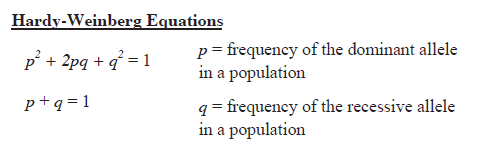# Hardy-Weinberg Study Guide

🗻 Big Picture: "In the absence of any evolutionary influences from generation to generation, the genotype and allele frequencies in a large, random-mating population remain constant." Examples of impacts include natural selection, genetic drift, mutation, sexual selection, gene flow, founder effect, meiotic drive, population bottleneck, inbreeding, and assortative mating." This is the statement of Hardy Weinberg law.

Check out awesome, educational VR rooms on Inspirit's mobile app (available for iOS and Android devices) 🤩

## Introduction

The Hardy-Weinberg principle states that genetic variance in a population will remain stable across generations in the absence of evolutionary forces. In other words, the rule states that if the frequency of distinct alleles in a population is already known, it is feasible to predict the anticipated frequencies of genotypes in a population under a restricted set of assumptions.

### Hardy Weinberg Equation

The two equations of the Hardy-Weinberg principle are represented below:Source

• 'p2' denotes the homozygous dominant genotype (AA)

• '2pq' denotes the heterozygous genotype (Aa)

• 'q2' denotes the homozygous recessive genotype (aa).

### Conditions of Hardy-Weinberg Equilibrium

Five conditions must be met for a population to reach Hardy-Weinberg equilibrium:

• No mutations in DNA

• No migration into or out of a population

• A significantly large population size

• Random mating

• Natural selection does not occur

If any of these five assumptions are broken, the population will still have the Hardy–Weinberg proportions at each generation, but the allele frequencies will shift over time.

### Applications of Hardy-Weinberg Principle

The Hardy-Weinberg law is a mathematical criterion that may compare non-evolving populations to evolving populations. If allele frequencies are tracked throughout time and anticipated frequencies are approximated using Hardy-Weinberg law values, then workings that drive population evolution may be theorized.

The rule offers a blueprint for studying the population genetics of diploid species that fulfill the fundamental requirements of random mating, large populations, no mutation, migration, or selection.

## ✅ Conclusion

• The Hardy-Weinberg principle asserts that allele frequencies within a population will remain stable over generations if the following criteria is met: no mutation, no migration, no natural selection, random mating, and a large population size.

• The first Hardy-Weinberg equation is (p² + 2pq + q² = 1), where 'p2' denotes the homozygous dominant genotype (AA), '2pq' denotes the heterozygous genotype (Aa), and 'q2' denotes the homozygous recessive genotype (aa).

• The second Hardy-Weinberg equation is (p + q = 1), where 'p' represents the frequency of a dominant allele in a population, and q represents the frequency of a recessive allele in a population.

• The frequency of alleles in a population may be calculated using this equation.

• The Hardy-Weinberg principle is useful for studying the population genetics of diploid species and theorizing what factors drive the evolution of a population.

### FAQs

1. What are the 5 conditions of the Hardy-Weinberg principle?

No mutation, no gene flow, a high population size, random mating, and no natural selection is required to preserve the Hardy-Weinberg equilibrium.

2. What is the Hardy Weinberg principle?

In the absence of disrupting events, the Hardy-Weinberg equilibrium states that genetic variation in a population will remain constant from generation to generation.

3. What are the 2 Hardy Weinberg equations?

• (p² 2pq q² = 1), where 'p2' denotes the homozygous dominant genotype (AA), '2pq' denotes the heterozygous genotype (Aa), and 'q2' denotes the homozygous recessive genotype (aa)

• (p + q = 1), where 'p' represents the frequency of a dominant allele in a population, and q represents the frequency of a recessive allele in a population.

4. What does the Hardy Weinberg equation tell us?

The Hardy-Weinberg equation is a mathematical formula for calculating the genetic variation of an equilibrium population. The Hardy-Weinberg equation may be used to compute the frequencies of the three genotypes if the p and q allele frequencies are known.

5. What is the purpose of Hardy Weinberg equilibrium?

Hardy-Weinberg Equilibrium (HWE) is a method for estimating the number of homozygous and heterozygous variant carriers in non-evolving populations based on allele frequency.

6. What is the difference between allele and genotype frequency?

The relative frequency of an allele on a genetic locus in a population is measured by allele or gene frequency. The proportion of a specific genotype among all individuals in a community is known as genotypic frequency.

We hope you enjoyed studying this lesson and learned something cool about the Hardy-Weinberg equation! Join our Discord community to get any questions you may have answered and to engage with other students just like you! Don't forget to download our App to experience our fun, VR classrooms - we promise, it makes studying much more fun! 😎

## Sources

1. Hardy–Weinberg principle https://en.wikipedia.org/wiki/Hardy%E2%80%93Weinberg_principle Accessed on 7 Dec 2021

2. Hardy-Weinberg equilibrium https://www.nature.com/scitable/definition/hardy-weinberg-equilibrium-122/ Accessed on 7 Dec 2021

3. Hardy-Weinberg law https://www.britannica.com/science/Hardy-Weinberg-law Accessed on 7 Dec 2021

4. Hardy-Weinberg Equilibrium http://www.germanna.edu/wp-content/uploads/tutoring/handouts/Hardy-Weinberg-Equilibrium.pdf Accessed on 7 Dec 2021